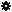﻿ Object Model: Edge.NeighborPolygons

Edge.NeighborPolygons

## Edge.NeighborPolygonsv3.0

### Description

Returns a collection of PolygonFace objects for all polygon neighbors within a given distance. If the distance is 1 the adjacent PolygonFace are returned (1 if border edge, 2 otherwise).

### C# Syntax

 ```PolygonFaceCollection Edge.NeighborPolygons( Int32 in_lDistance ); ```

### Scripting Syntax

 ```oReturn = Edge.NeighborPolygons( [Distance] ); ```

### Parameters

Parameter Type Description
Distance Integer Distance is an integer value representing the degree of neighborhood (for example, degree=2 for a polygon means its adjacent polygons plus the adjacent polygons of the adjacent polygons).

Default Value: 1

### Examples

#### VBScript Example

 ```set oCube = ActiveSceneRoot.AddGeometry( "Cube", "MeshSurface" ) set oGeometry = oCube.ActivePrimitive.Geometry for each Edge in oGeometry.Edges set oNeighbors = Edge.NeighborPolygons( 1 ) str = "Polygons neighbors of segment( " & Edge.index & ") are :" for each n in oNeighbors str = str & " " & n.index next logmessage str next ```